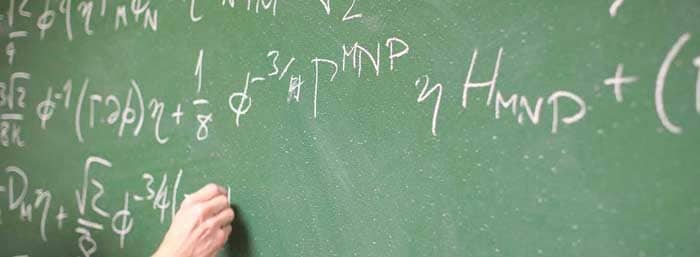# Centre of Mass problems with answers### Centre of mass questions:

Ques. A bomb travelling in a parabolic path under the effect of gravity explodes in mid air. The centre of mass of fragments will
(a) Move vertically upwards and then vertically downwards
(b) Move vertically upwards
(c) Move in an irregular path
(d) Move in the parabolic path the unexploded bomb would have travelled
Ans. (d)

Related: Biotechnology mcqs online test

Ques. The centre of mass of the system of particles does not depend on
(a) Masses of the particles
(b) Forces on the particles
(c) Position of the particles
(d) Relative distance between the particles
Ans. (b)

Ques. The centre of mass of a body:
(a) Lies always at the geometrical centre
(b) Lies always inside the body
(c) Lies always outside the body
(d) May lie within or outside the body
Ans. (d)

Related: Thermodynamics physics questions

Ques. A system consists of two masses M and m(<M).  The centre of mass of the system is:
(a) at the middle
(b) nearer to M
(c) nearer to m
(d) at the position of small mass
Ans. (b)

Ques. Two particles of mass 1kg and 2kg are located at x = 0 and x = 3m. Find the position of their centre of mass.
(a) 2m
(b) 4m
(c) 6m
(d) 8m
Ans. (a)

Related: Biology main branches

Ques. In equilibrium, the Potassium and Iodine atoms of KI are separated by 3.0×10–10 m.  Potassium has a mass of 40.0 mass units and iodine has 120 mass units. How far from the K atom is the centre of mass of this molecule located?
(a) 2.25 x 1010 m
(b) 3.25 x 1010 m
(c) 2.65 x 1010 m
(d) 3.2 x 1010 m
Ans. (a)

Ques. Mass of a ring is non-uniformly distributed around its geometric centre.  If R is radius of the ring, then
(a) Centre of mass does not coincide with geomtic centre.
(b) Position of centre of mass from the geometric centre will be x (0 < x < R).
(c) Centre of mass will be nearer to the greater mass distribution.
(d) All of these
Ans. (d)

Related: Laws of motion questions

Ques. If the external forces acting on a system have zero resultant, the centre of mass
(a) must not move
(b) must not accelerate
(c) may move
(d) may accelerate.
Ans. (b) and (c)

Ques. Two balls are thrown simultaneously in air. The acceleration of the centre of mass of the two balls while in air
(a) depends on the direction of the motion of the balls
(b) depends on the masses of the two balls
(c) depends on the speeds of the two balls
(d) is equal to g
Ans. (d)

### About the author

#### Richa Bhardwaj

Richa (B. Tech) has keen interest in Science and loves to teach students about it through lectures and assignments. She always try to use simple language and sentences while writing to make sure learner understands everything properly.